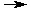Performing Titrations

## Performing Titrations

The most common use of titrations is for determining the unknown concentration of a component (the analyte) in a solution by reacting it with a solution of another compound (the titrant). From the known concentration of the titrant, the volume of titrant added and the stoichiometry of the reaction, the concentration of the analyte can be determined.

While there are many different types of titrations, acid-base titrations are the most common. Consider this example in which a sample of hydrochloric acid (HCl) is titrated with sodium hydroxide (NaOH).

HCl + NaOHNaCl + H2O

During the course of the titration, the titrant (NaOH) is added slowly to the unknown solution. As it is added, the HCl is slowly reacted away. The point at which exactly enough titrant (NaOH) has been added to react with all of the analyte (HCl) is called the equivalence point. Up to the equivalence point, the solution will be acidic because excess HCl remains in the flask. After the equivalence point, there will be an excess of NaOH and the solution will be basic.

An acid-base indicator can be used to help identify when the change from acidic to basic occurs. Phenolphthalein is colorless in acidic solutions and bright purple in basic solutions. As such, it should change from colorless to purple around the equivalence point of this titration. Our approximation of where the equivalence point occurs is called the endpoint. Care must be exercised when an indicator is chosen for a titration to ensure that the endpoint coincides as closely with the equivalence point as possible.

The individual steps required to perform this kind of titration are described on the next page.